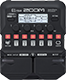# Cap'n Crunch

Discussion in 'Zoom G1/G1X Four' started by YellowWaits, Feb 20, 2020.

1. Cap'n CrunchDevice: Zoom G1 Four
Firmware: 1.00

Name on device: FD-TS-CRNCH
Optimized for: Phones/Speaker

Effects chain:Nice Rock'n'Roll overdriven sound.

Effect: "TS Drive" (Overdrive / Distortion), active - "yes"
"Gain" = 52
"Boost" = On
"Tone" = 62
"Volume" = 86

Effect: "Gt GEQ 7" (Filter), active - "yes"
"100" = 5.0
"200" = 0.5
"400" = 0.0
"800" = 6.0
"1.6k" = 2.5
"3.2k" = -6.5
"6.4k" = -4.0
"VOL" = 80

Effect: "HD Hall" (Reverb), active - "yes"
"PreD" = 63
"Decay" = 22
"Mix" = 14
"Tail" = Off

Effect: "FD TWNR" (Amp simulator), active - "yes"
"Bass" = 41
"Middle" = 42
"Treble" = 56
"Bright" = ON
"Gain" = 32
"Volume" = 59
"Depth" = 10
"Speed" = 10

Effect: "FD2x12" (Cabinet), active - "yes"
"MIC" = ON
"D57: D421" = 50
"Hi" = 50
"Lo" = 50

Patch Volume: 100